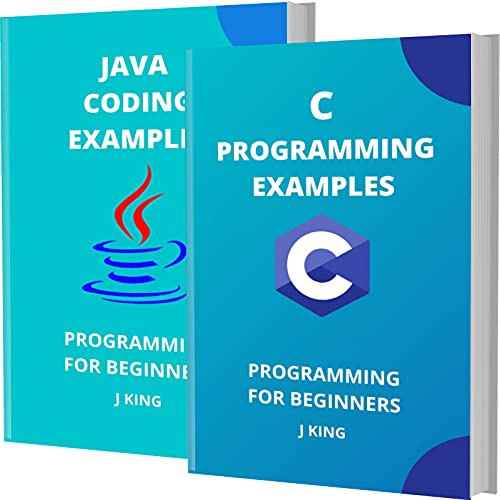# C AND JAVA CODING EXAMPLES: PROGRAMMING FOR BEGINNERS

by
• Length: 91 pages
• Edition: 1
• Publication Date: 2021-05-08
• ISBN-10: B094HH99NF
Description

YOU WILL SAVE 33% WITH THIS OFFER.
This Books Absolutely For Beginners:
You can learn primary skills of C AND JAVA PROGRAMMING fast and easily. The book includes practical examples for beginners.

TABLE OF CONTENTS
C program to find SUM and AVERAGE of two numbers.
C program to print ASCII value of a character
C program to print all numbers from 1 to N using goto statement
Program to print numbers from 1 to N using while loop
Program to print Square, Cube and Square Root
Program to print table of a given number
Program for Largest Number among three numbers
Area of rectangle program in c
C program – Convert Feet to Inches
Program to create a text file using file handling
C program to find factorial of a number
Program to swap two bits of a byte
Program to swap two numbers using four different methods
Age Calculator (C program to calculate age)
C program to design a digital clock
Program to calculate compound interest
Program to check whether number is Perfect Square or not
Program to print message without using any semicolon in program
Sum of Series Programs / Examples using C
JAVA CODING EXAMPLES
Java program to print ‘Hello world’
Print different type of values in Java
Read and print an integer value in Java
Java program to find sum and average
Java program to print Christmas tree
Java program to find sum of all digits
Java program to calculate compound interest
Java program to find largest number
Java program to run an application
Java program to print Fibonacci Series
Using for loop to print numbers from 1 to N
Using while loop to print numbers from 1 to N
Addition of one dimensional and two dimensional arrays
Java program to convert Decimal to Binary
Java program to read a file line by line
Java program to get current system date and time
Java program to Calculate Area of a Circle.
Java – Print File Content, Display File
Java program to copy files
Find sum of factorials from 1 to N
Find smallest element in an array

To access the link, solve the captcha.